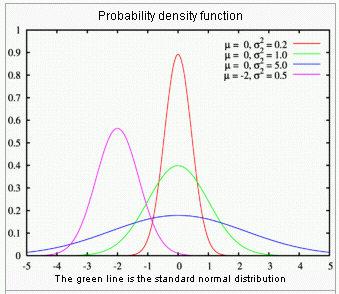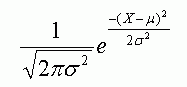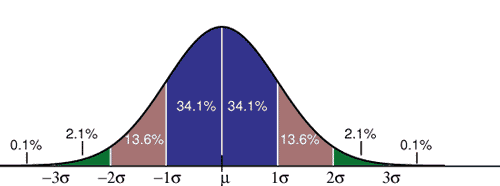Email us to get an instant 20% discount on highly effective K-12 Math & English kwizNET Programs!

#### Online Quiz (WorksheetABCD)

Questions Per Quiz = 2 4 6 8 10

### Middle/High School Algebra, Geometry, and Statistics (AGS)9.28 Normal or Gaussian Distributions

#### Probability Distribution

A probability distribution describes the values and probabilities that a random event can take place. The values must cover all of the possible outcomes of the event, while the total probabilities must sum to exactly 1, or 100%. For example, a single coin flip can take values Heads or Tails with a probability of exactly 1/2 for each; these two values and two probabilities make up the probability distribution of the single coin flipping event.

#### Normal DistributionsNormal distributions, also called Gaussian distributions, are a family of probability distributions that have the same general shape. All normal distributions are symmetric and have bell-shaped density curves with a single peak.

The normal distributions are a very important class of statistical distributions. Many psychological measurements and physical phenomena (like noise) can be approximated well by the normal distribution.

#### Characteristics of Normal Distributions

• Each member of the family may be defined by two parameters, location and scale: the mean (m) and variance or square of standard deviation (s2), respectively. The standard normal distribution is the normal distribution with a mean of zero and a variance of one.

• In a normal distribution, the mean m (mu) indicates the location of the peak density, and the standard deviation s (sigma), indicates the spread or girth of the curve.

• Normal distributions differ in how spread out they are, but the area under each curve is the same.

• Mathematical formula for a normal distribution is:where,

• m - mean
• s - standard deviation
• p - constant 3.14159
• e - is the base of natural logarithms = 2.718282
• X can take on any value from -infinity to + infinity

• Different values of m and s yield different normal density curves and hence different normal distributions.

• About 68% of values drawn from a normal distribution are within one standard deviation away from the mean; about 95% of the values are within two standard deviations and about 99.7% lie within three standard deviations (see picture below).#### The Standard Normal Distribution or Z Distribution

• The standard normal distribution is a normal distributions with a mean of 0 and a standard deviation of 1. Normal distributions can be transformed to standard distributions by the formula:

Z = X - m/s

Where X is a score from the original normal distribution, m is the mean of the original normal distribution, and s is the standard deviation of original normal distribution.

The standard normal distribution is sometimes called the Z distribution. A 'Z score' always reflects the number of standard deviations above or below the mean a particular score is. For instance, if a person scored a 70 on a test with a mean of 50 and a standard deviation of 10, then he scored 2 standard deviations above the mean. Converting the test scores to Z scores, an X of 70 would be:

Z = 70-50/10 = 2

So, a Z score of 2 means the original score was 2 standard deviations above the mean. Note that the Z distribution will only be a normal distribution if the original distribution (X) is normal.

• What is a probability distribution?
• Define normal distribution and give its significance.
• What is a standard normal distribution? Give examples.

•  Q 1: If scores are normally distributed with a mean of 30 and a standard deviation of 5, about what percent of the scores are between 28 and 34? 66334488 Q 2: The normal distribution is defined by two parameters, they are ______ and ________.mean and standard deviationmean and modevariance and standard deviationmean and z-value Q 3: The standard normal distribution is also calledZ distributionskewed distributionsquare distributiondiscrete distribution Q 4: What proportion of a normal distribution is within one standard deviation of the mean? 0.4530.5320.6820.999 Q 5: What proportion is between 1 and 1.5 standard deviations above the mean?0.1320.0910.3450.456 Q 6: All normal distributions are symmetric and have ____ shaped density curves with a single peak. randomuniformsquarebell Question 7: This question is available to subscribers only! Question 8: This question is available to subscribers only!